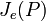# ZJe-subgroup

Jump to: navigation, search
This article defines a subgroup-defining function, viz., a rule that takes a group and outputs a unique subgroup
View a complete list of subgroup-defining functions OR View a complete list of quotient-defining functions

## Definition

Let$p$ be a prime number and$P$ be a finite p-group. The ZJe-subgroup of$P$, denoted$Z(J_e(P))$, is defined as the center of the join of elementary abelian subgroups of maximum order (denoted$J_e(P)$). It is related to, but not necessarily the same as, the ZJ-subgroup, which is the center of the join of abelian subgroups of maximum order (denoted$J(P)$).$J_e(P)$ and$J(P)$ are two of the Thompson subgroups.